Scattering and tunnelling

Start this free course now. Just create an account and sign in. Enrol and complete the course for a free statement of participation or digital badge if available.

Free course

# 4.4 Stationary states and tunnelling in one dimension

We will now use the stationary-state approach to analyse the tunnelling of particles of energy E0 through a finite square barrier of width L and height V0 when E0 < V0 (see Figure 21).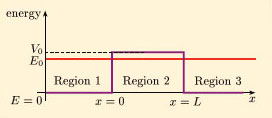Figure 21 A finite square barrier of width L and height V0, together with the energy E0 of each tunnelling particle

Our main aim will be to find an expression for the transmission coefficient. By now, you should be familiar with the general technique for dealing with problems of this kind, including the existence of exponentially growing and decaying solutions in the classically forbidden region, so we shall immediately write down the solution of the relevant time-independent Schrödinger equation: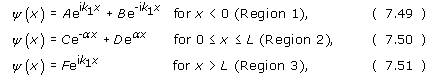where A, B, C, D and F are arbitrary constants and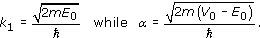Note that the term that might describe a leftward moving beam in Region 3 has already been omitted, and the wave number in Region 3 has been set equal to that in Region 1.

Requiring the continuity of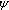(x) and d/dx at x = 0 and at x = L leads to the following four relations: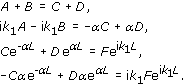After some lengthy algebra, similar to that in Worked Example 1, these four equations can be reduced to a relationship between F and A, from which it is possible to obtain the following expression for the transmission coefficient T = |F/A|2.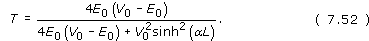When αL ≫ 1, the denominator of this expression can be approximated by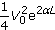, and the transmission coefficient through the barrier is well-described by the useful relationship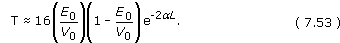(Remember: sinh x = (exe−x)/2.)

This shows the exponential behaviour that might have been expected on the basis of our earlier results for barrier penetration into a square step, but in this case it is a true tunnelling result. It tells us that tunnelling will occur, but indicates that the tunnelling probability will generally be rather small when αL ≫ 1, and will decrease rapidly as the barrier width L increases.

A graph of |2| plotted against x for a finite square barrier in the case E0 < V0 will look something like Figure 22. Note that because the incident and reflected beams have different intensities, the minimum value of |2| in Region 1 is always greater than zero. Also note that for a square barrier of finite width the declining curve in Region 2 is not described by a simple exponential function; there are both exponentially decreasing and exponentially increasing contributions to(x) in that region.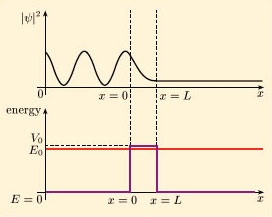Figure 22 The quantity |ψ2| plotted against x for a finite square barrier in the case E0 < V0

## Worked Example 2

Electrons with a kinetic energy of 5 eV are incident upon a finite square barrier with a height of 10 eV and a width of 0.5 nm. Estimate the value of T and hence the probability that any particular electron will tunnel through the barrier.

### Solution

In this case, V0E0 = (10 − 5) eV = 5 × 1.6 × 10−19J. It follows that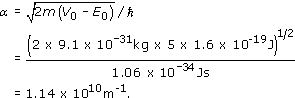With L = 5 × 10−10 m, it follows that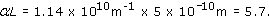Since this is much larger than 1, we can use Equation 7.53 to estimate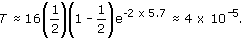This is the probability that any particular electron will tunnel through the barrier.

## Exercise 12

Determine the probability current in each of the three regions in the case of tunnelling through a finite square barrier. Comment on the significance of your result for Region 2.

From Equation 7.29,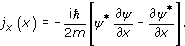In Region 1, where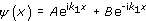and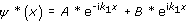, we have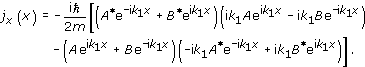Simplifying gives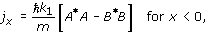which can be interpreted as the sum of the probability density currents associated with the incident and reflected beams.

Similarly, in Region 2, where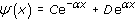and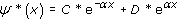, we have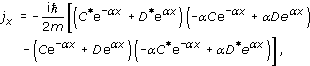which simplifies to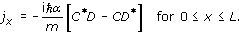Finally, in Region 3, where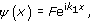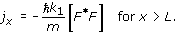The result for Region 2 is a real non-zero quantity, so there is a probability current inside the barrier. This is not really surprising since particles must pass through the barrier to produce a transmitted beam in Region 3. In fact, conservation of probability requires that the probability current should be the same in all three regions.

SM358_1